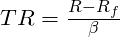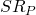Warning: "continue" targeting switch is equivalent to "break". Did you mean to use "continue 2"? in /home/calcul9/public_html/wp-content/themes/suffusion/functions/media.php on line 580

Warning: "continue" targeting switch is equivalent to "break". Did you mean to use "continue 2"? in /home/calcul9/public_html/wp-content/themes/suffusion/functions/media.php on line 583

Warning: "continue" targeting switch is equivalent to "break". Did you mean to use "continue 2"? in /home/calcul9/public_html/wp-content/themes/suffusion/functions/media.php on line 586

Warning: "continue" targeting switch is equivalent to "break". Did you mean to use "continue 2"? in /home/calcul9/public_html/wp-content/themes/suffusion/functions/media.php on line 589

Warning: "continue" targeting switch is equivalent to "break". Did you mean to use "continue 2"? in /home/calcul9/public_html/wp-content/themes/suffusion/functions/media.php on line 592
Performance Ratios: The Sharpe Ratio and Beyond » The Calculating Investor

# Performance Ratios: The Sharpe Ratio and Beyond

Apr 122011

#### Evaluating Investment Alternatives Using Performance Ratios

In this post, I look at several ratios which are used to evaluate investment performance.

The Sharpe ratio, Treynor ratio, and information ratio are all common ratios for evaluating investment managers and investment portfolios.  Each of these measures can be used ex post to evaluate past performance, or they can be used ex ante to help investors make portfolio decisions based on forecasts for various investment alternatives.

The Sharpe Ratio

The Sharpe ratio is one of the most common metrics for evaluating portfolios.

The Sharpe ratio is calculated by dividing the mean excess return of the portfolio by the standard deviation of the excess return.  In other words, this ratio measures the “reward” we can get for a particular level of variability or  “risk”.

The equation for calculating the Sharpe ratio is:Bigger is better for the Sharpe ratio.  High-reward and low-risk results in a relatively large Sharpe ratio, and low-reward and high-risk results in a relatively small Sharpe ratio.

The Sharpe ratio is typically calculated using annualized return and standard deviation, but it can be calculated for other time intervals.

The Sharpe ratio is sometimes calculated using total returns rather than excess returns, but this is incorrect.  It is important to calculate the Sharpe Ratio using excess returns.

As an example, consider two assets, A and B.  Asset A has a total return of 6% and a standard deviation of 12%. Asset B has a total return of 9% and a standard deviation of 20%. The return-to-standard-deviation ratio is 0.5 for Asset A, and 0.45 for Asset B.  Based on these ratios, we might conclude that Asset A is the superior investment.

However, if we assume a risk-free rate of 3%, then the true Sharpe ratio calculation gives a different answer.  If we properly deduct the risk-free rate from the total returns, we find that the Sharpe ratio of Asset A is 0.25 and the Sharpe ratio of Asset B is 0.30. Therefore, the Sharpe ratio suggests that Asset B is the superior investment.

Which calculation method gives the correct answer? The correct answer is given by the Sharpe ratio.  For example, if we wanted to target a standard deviation of 12%, we could invest 100% of our funds in Asset A or we could invest 60% in Asset B and 40% in the risk-free asset.  The first option, using Asset A, gives a total return of 6% with a standard deviation of 12%.  The second option, using Asset B and the risk-free asset, gives a total return of 6.6% with a standard deviation of 12%.  Clearly, the portfolio using Asset B and the risk-free asset will provide a higher return with lower risk, and this is consistent with Asset B having a higher Sharpe ratio.

The Treynor Ratio

The Treynor ratio is similar to the Sharpe ratio, but CAPM beta is used in the denominator rather than the standard deviation.  This is an important difference.  Since the Treynor ratio uses only the non-diversifiable risk as the denominator, it should be used for evaluating funds or assets which are being added to a portfolio which is already well diversified.

The Treynor ratio is calculated using this equation:As with the Sharpe ratio, a larger Treynor ratio is better, and the ratio is typically calculated using annualized returns and standard deviations.

The Information Ratio

The information ratio is used to quantify the value added by active investment managers.

The information ratio is the “alpha” of a portfolio divided by the tracking error.   In other words, the information ratio is the excess return relative to a benchmark that a manager generates divided by the extra risk that the manager takes on in order to generate that excess return.

The information ratio is calculated using this equation:The information ratio closely related to the Sharpe ratio.  If we believe that we can identify active managers with a persistent and predictable level of skill (a big “if” in my opinion), then the information ratio helps us to incorporate this skill into a portfolio in a manner which maximizes the  Sharpe ratio.

For example, if we have an active portfolio with an information ratio equal toand a market index with a Sharpe ratio equal to, then the maximum portfolio Sharpe ratio () is given by this equation:There is some additional algebra required to determine the optimal weight to assign to the index and active portfolio to achieve this Sharpe ratio, but that is beyond the scope of this post.  For investors who are interested in learning more about how the information ratio can be used to optimize active portfolios, I recommend the book Active Portfolio Management by Grinold and Kahn.

### 6 Responses to “Performance Ratios: The Sharpe Ratio and Beyond”

1.Excellent overview. One place to find the statistics is at Yahoo Finance. For example, put xle (energy etf) in the quote box. Scroll down and on the RHS find a link for “risk details”:

http://finance.yahoo.com/q/rk?s=XLE

Here you’ll find the Sharpe ratio based on various time spans of data and other metrics.

•Thanks, that is a good tip! I wasn’t aware that Yahoo Finance provided the Sharpe ratio stats.

2.I tend to use a mixture of the Sharpe and Sortino ratio to decide where to invest (and to backtest my portfolio). I hardly ever look at raw investment returns in isolation (they can be very misleading). There’s an Excel spreadsheet for calculating the Sharpe Ratio at http://optimizeyourportfolio.blogspot.com/2011/05/calculating-sharpe-ratio-with-excel.html

3.I’ve started to use the Omega Ratio as my “go to” performance benchmark because it addressess the shortcomings of traditional benchmarks like the Sharpe or Sortino Ratio.

For example, the Sharpe Ratio approximates the returns distribution with the mean and standard deviation, and assumes the returns follow a normal distribution. Its one primary advantage is that everyone uses it.

The Omega Ratio effectively captures all of the information within the empirical returns distribution (and distinguishes between downside and upside risk). However, it’s not as widely used as the Sharpe Ratio, so it’s usually not given in mutual fund factsheets etc. I used the Omega Ratio Excel spreadsheet here to calculate the Omega Ratio myself.

4.I have a question on Standard Deviation when using the Sharpe Ratio. That is, the Std Dev of what, exactly?

Is it the Std Dev of returns? Or Std Dev of the account value?

I assume it’s the Std Dev of the account value, but I’m finding it difficult to get confirmation anywhere. And it makes a HUGE difference to the final Sharpe ratio.

What is the standard? Any help would be appreciated…

•The standard deviation of the excess return of the asset or portfolio.